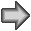Aptitude Tests 4 Me

Non Verbal Logic

A. Series completion: A common approach can be evolved to solve the series completion type questions because the underlying principle is almost similar in all the cases. The various relationship that govern the series completion category of questions can be

1. Number of dots in successive figures increasing by one.

2. The geometrical figure inside each circle changing with the successive elimination of one side.

3. The bars inside the geometrical figures increasing by one.

4. The geometrical figure rotating clockwise or anti clockwise.

5. The blank circle appearing alternatively in successive figures.

6. The arcs changing curvature in alternative figures.

7. The line on a circle moving clockwise or anticlockwise.

8. The symbols moving clockwise or anticlockwise.

B. Analogies: Here two sets of problem figures are given. One set of problem figure has certain analogy between them. Based on this we have to complete the second figure of the second set. The various types of analogies could be

1. The arrow touching the circle in the opposite direction.

2. The bar '-' rotating about its axis.

3. The number of sides reducing or increasing by half.

4. The number of lines decreasing or increasing by half.

5. The number of circles decreasing or increasing by half or one third.

6. The second figure being mirror image of the first.

7. Number of sided decreasing by 1 and the alphabets in the corners correspond to the numbers.

8. The figure rotating in the clockwise or anti clockwise direction.

9. The figures inside the rectangle is inverted and the symbols surrounding it move in clockwise or anti clockwise direction.

10. One arrow is added in each block

11. The arrow points outwards. The small circles change to arcs and the bracket turns upwards.

12. The oval becomes a rectange and the symbols interchange position.

C. Odd Man Out: Here four figures are given and one of them is the answer figure i.e, the three are similar in some fashion and the fourth one i.e, the correct answer is the odd man out. The various type of situations could be

1. Each element rottes in a clockwise direction in all the figures except the correct answer i.e, the odd man out.

2. The '+' and dot rotate in anti clockwise direction in all the figures except the correct answer i.e, the odd man out.

3. There are two or more intersections in all the figures except the correct answer i.e, the odd man out.

4. The elements follow a fixed pattern in all the figures except the correct answer i.e, the odd man out.

5. The figure rotates in a clockwise direction in all the figures except the correct answer i.e, the odd man out.

6. The line segment intersecting the circular region in only figure.

7. Number of dots inside the triangle is equal to those outside in only one figure.

Thus, in non verbal logical question you are required to establish some definite relationship between thes figures and then apply it to the new situation. The types of question set can be divided into three categoreis (1) Series, (2) Analogy, (3) Classification.

Series: In these types of questions there is a systematic change occuring from left to right in the Problem figure. You are required to find the nature on this change and then decide what will be the next figure from the answer figures subject to the same change. These changes can be of different types and are discussed below:

1. Additions, Deletions and Replacements of Elements

2. Rotational and Movements of Elements

3. Rearrangements/Rotation of Elements

5. Deletion of Elements

6. Movements of Elements/Similar Movements

7. Changes in Elements/Movements of Elements

8. Rotations/Deletions of Elements

9. Changing the Positions of elements/Replacements of Elements

10. Movements of Elements/Identical figures

11. Interchanging the Positions of Elements alongwith Change in Elements

12. Changes in Elements / Movements of Elements

13. Rotations/Movements/Replacements of Elements

14. Movements of Elements/Changes in Elements

15. Identical Figures

16. Rotations/Replacements/Interchanging the Position of Elements

Analogy: In this type problem figures are presented in two units. the first unit contains two figures, one in each square. The second unit contains one figure in the first square and a question mark (?) in the second square. You have to find out from among the given four options as to which should replace the question mark after finding the relationship between the two figures in the first unit of the problem figures.

Classification: In this type four figures numbered 1, 2, 3, and 4 are given. These are treated both as problem figures as well as the answer figures. Three out of these four figures are related to each other by way of having some common characteristics and so form a group. Out of these four, you have to identify one figure which does not belong to that group.

In the next 88 pages you will find 310 questions on non verbal logic or visual reasoning and their solutions with detailed explanation.1 2 3 4 5 6 7 8 9 10 11 12 13 14 15 16 17 18 19 20 21 22 23 24 25 26 27 28 29 30 31 32 33 34 35 36 37 38 39 40 41 42 43 44 45 46 47 48 49 50 51 52 53 54 55 56 57 58 59 60 61 62 63 64 65 66 67 68 69 70 71 72 73 74 75 76 77 78 79 80 81 82 83 84 85 86 87 88Passage ReadingVerbal LogicNon Verbal LogicNumerical LogicData InterpretationReasoningAnalytical AbilityBasic NumeracyAbout UsContactPrivacy PolicyMajor TestsFAQ